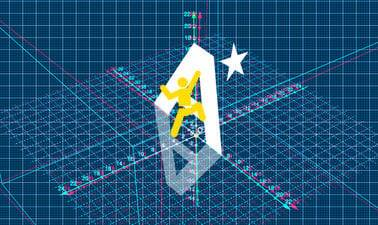# Further Mathematics Year 13 course 2: Applications of Differential Equations, Momentum, Work, Energy & Power, The Poisson Distribution, The Central Limit Theorem, Chi Squared Tests, Type I and II ErrorsThis course by Imperial College London is designed to help you develop the skills you need to succeed in your A-level further maths exams. You will investigate key topic areas to gain a deeper understanding of the skills and techniques that you can apply throughout your A-level study. These skills include: * Fluency – selecting and applying correct methods to answer with speed and efficiency * Confidence – critically assessing mathematical methods and investigating ways to apply them * Problem solving – analysing the ‘unfamiliar’ and identifying which skills and techniques you require to answer questions * Constructing mathematical argument – using mathematical tools such as diagrams, graphs, logical deduction, mathematical symbols, mathematical language, construct mathematical argument and present precisely to others * Deep reasoning – analysing and critiquing mathematical techniques, arguments, formulae and proofs to comprehend how they can be applied Over eight modules, you will be introduced to * Simple harmonic motion and damped oscillations. * Impulse and momentum. * The work done by a constant and a variable force, kinetic and potential energy (both gravitational and elastic) conservation of energy, the work-energy principle, conservative and dissipative forces, power. * Oblique impact for elastic and inelastic collision in two dimensions. * The Poisson distribution, its properties, approximation to a binomial distribution and hypothesis testing. * The distribution of sample means and the central limit theorem. * Chi-squared tests, contingency tables, fitting a theoretical distribution and goodness of fit. * Type I and type II errors in statistical tests. Your initial skillset will be extended to give a clear understanding of how background knowledge underpins the A -level further mathematics course. You’ll also be encouraged to consider how what you know fits into the wider mathematical world.

Created by: Imperial College London

Level: IntermediateNote: must be in .png, .gif or .jpg format
ORNote: must be in .png, .gif or .jpg format

By clicking this button,